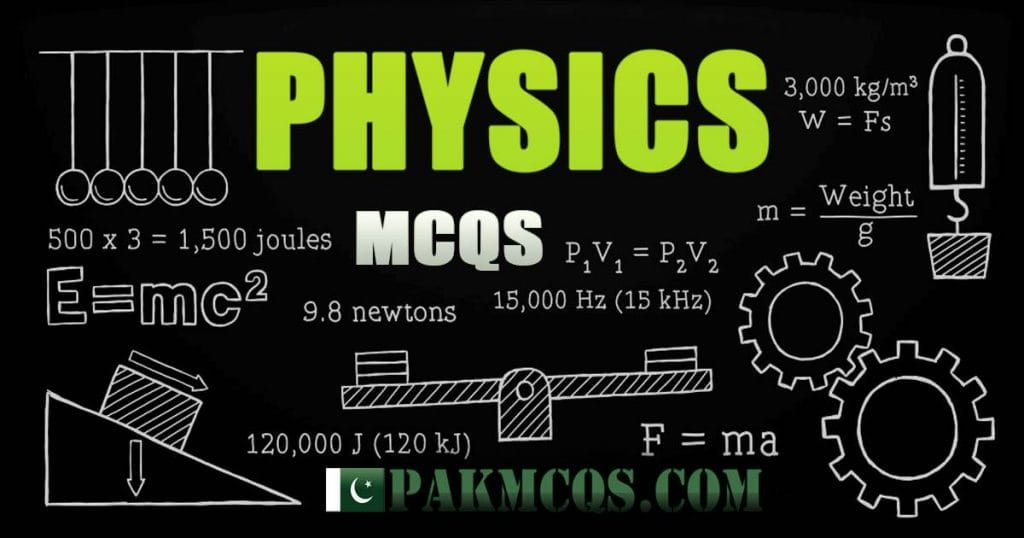A. 2.5
B. 2.0
C. 1.75
D. 1.5
E. 1.25

Submitted by: Mansoor Ul Haque

One moves at 60km/h, so in an hour, he’ll travel 60km
The other moves at 40km/h, so in an hour, he’ll travel 40km
This is towards each other, so, in an hour, we can say that they’ll get 60+40=100km closer.

This means that they get closer at 100km/h

Given that we have the distance of 150km, and the speed of closure at 100kmh, we can now use the equation

S=D/T

Rearrange to find T (times both sides by T)

S x T = D (now divide both sides by S)

T=D/S

Time = 150km divided by 100km/h

Time = 1.5 hours

Or one hour and 30 minutes

Arithmetic Mcqs

Basic Arithmetic Mcqs for the Prepration of FPSC Tests, PSC Tests, NTS Test. Important Arithmetic Aptitude Mcqs questions. Important Arithmetic Aptitude Mcqs questions. average, percentage, Ratio, etc. some of the most important sub categories are: Average, Percentage, Problem on Ages, Time and Distance, HCF and LCM, Logarithms, Discount, Interest, Ratio & Proportion, Decimal Fraction and other.

BASIC ARITHMETI MCQS
1. Percentage 8. Average
2. Profit and Loss 9. Time & Distance
3. Discount 10. Work-Time
4. Stocks and Shares 11. Men Food
5. interest 12. Height and Distance
6. Odd Man Out and Series 13. Volume and Surface Area
7. Ratio 14. Time and Work
Mathematics McqsMathematics Mcqs - Maths Mcqs are very important for every test. prepare for NTS, FPSC, PPSC, SPSC, CSS, PMS Test Preparation. and all other testing services. Most of the test syllabus contain Mathematics test portion. Here you will find most important Mathematics Mcqs. here these Mcqs will help you getting good marks in this Section. Here you will find most important Mcqs of Mathematics from Basic to Advance. some of the most important sub categories are: Average, Percentage, Problem on Ages, Time and Distance, HCF and LCM, Logarithms, Discount, Interest, Ratio & Proportion, Decimal Fraction and other.

MATHEMATICS MCQS
1. Basic Maths Mcqs 17. Arithmetic Mcqs
2. Average Mcqs 18. Boats and Streams
3. Compound Interest 19. Areas
4. Height and Distance Mcqs 20. Discount Mcqs
5. Men Food Mcqs 21. interest Mcqs
6. Mixtures and Allegations 22. Mensuration
7. Partnership 23. Odd Man Out Series Mcqs
8. Pipes and Cisterns 24. Volumes
9. Permutations and Combinations 25. Probability
10. Problems on L.C.M and H.C.F 26. Problems on Numbers
11. Profit and Loss Mcqs 27. Quadratic Equations
12. Races and Games 28. Ratio and Proportion
13. Simple Equations 29. Simplification and Approximation
14. Stocks and Shares Mcqs 30. Time & Distance Mcqs
15. Time and Work Mcqs 31. Volume and Surface Area Mcqs
16. Percentage Mcqs 32. Math Solutions
Physics McqsPhysics Mcqs for Test Preparation from Basic to Advance. Physics Mcqs are from the different sections of Physics. Here you will find Mcqs of Physics subject from Basic to Advance. Which will help you to get higher marks in Physics subject. These Mcqs are useful for students and job seekers i.e MCAT ECAT ETEA test preparation, PPSC Test, FPSC Test, SPSC Test, KPPSC Test,BPSC Test, PTS ,OTS,GTS,JTS,CTS.Download Medical Entry Test App:
Time & Distance Mcqs
Time & Distance Mcqs calculations for Mathematics Preparation with detailed explanation.

IF YOU THINK THAT ABOVE POSTED MCQ IS WRONG.

###### 17 Comments to “Two automobiles are 150 kilometers apart and traveling toward each other. One automobile is moving at 60km/h and the other is moving at 40km/h mph. In how many hours will they meet?”
1. Aamir says:

2. Sonia shahid says:

Assalam-o-Alaikum. I had apply for the post of Bureau of Statistics BPS-17. MCQS or any Special tips that might be help full for my test that’s is going to held on 6th January.

3. ayat says:

4. zoni says:

Can u plz give the solution of this question

5. noor nabi says:

simple hai agar dono k km dekhe jay to 1 hour me dono 100 km cover kr rahe hain matlb remainig 50 km bach gay to agar 1 hour me 100 km agar kr rhe hain to to 50 km half an hour me honge means total hours 1.5 lage

6. Rab Nawaz says:

Bro ye maths hai to yahan pe answers k sath solutiom b hona chahye jese smjh aa sake prove zaruri hai to lehaaza solution b diya hai data k sath. thanks

7. Shoaib abro says:

Why eds portion contains arithmetic?

1. thanks for pointing it out. it has been moved to maths section

8. Akramullah khan says:

Respected sir,
Sir physics ki koi mcqs book ka naam batayen

9. Iman says:

Solution?

10. Zulqarnain saahi says:

Suppose time = x hours
Both cars will have same time, but different distances depending upon speed.
So,

(60km/h).x + (40km/h).x = 150
(100 km/hour ).x =150
X= 1.5

11. Dear I need past ppsc papers of lect physics
If you have plz share me
Will be thankful to you

12. Alam Zeb says:

Simple is one is moving with 60km/hr means
150/60 = 2.5 hr
150/40 = 3.75 the difference between both is 3.75-2.5 = 1.25

13. Ahmed Ali Khuwaj says:

Speed = Distance/time
t=d/s
T1=150/60 =2.5
T2=150/40= 3.75
Meeting time=T2-T1
=3.75-2.5
=1.25